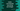# Kotlin program to concat one string and integer## Introduction :

Kotlin program to concat one string and one integer value. For example, if the string is ‘hello-’ and number is 20, it will print ‘hello-20’.

I will show you different ways to do that in Kotlin.

### Using plus() :

We can use plus() method of String class to concatenate one string with a number. It is defined as below :

``public operator fun plus(other: Any?): String``

This method takes one argument and adds it with the reference string and returns the new final string. If the argument is not string, it takes the string representation of the argument. In our case, we will pass one integer and it will be converted to a string and appends it to the reference string.

``````fun main() {
val str = "Hello-"
val num = 20

print(str.plus(num))
}``````

It will print :

``Hello-20.``

### Using + :

+ is another way to add one string with an integer. If we add two integers, it returns the sum. But if we add one string with an integer, it will concatenate the string with the integer and return the new string.

``````fun main() {
val str = "Hello-"
val num = 20

print(str + num)
}``````

It will print :

``Hello-20.``

### Using string template :

String templates are string literals that can also contain expressions. We can concatenate one string with an integer value using string templates like below :

``````fun main() {
val str = "Hello-"
val num = 20

print("\$str\$num")
}``````

### Conclusion :

Let’s put all above methods in one example :

``````fun main() {
val str = "Hello-"
val num = 20

println(str.plus(num))
println(str + num)
println("\$str\$num")
}``````